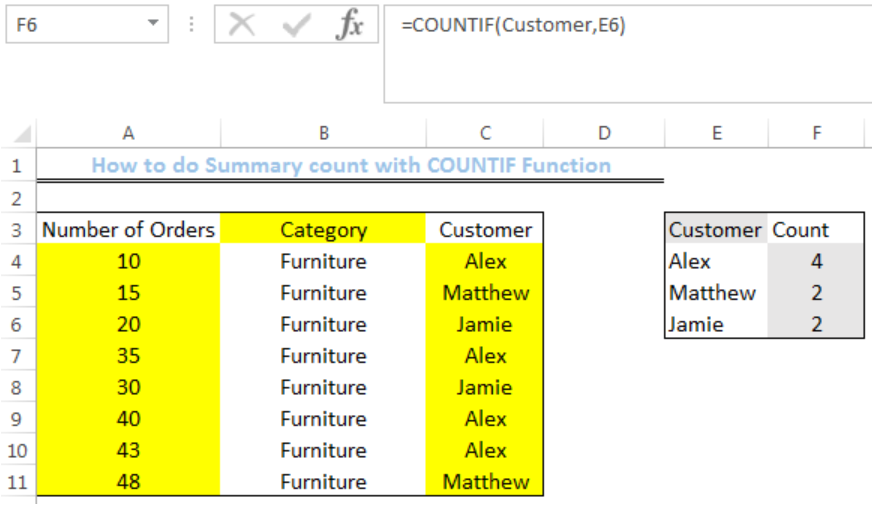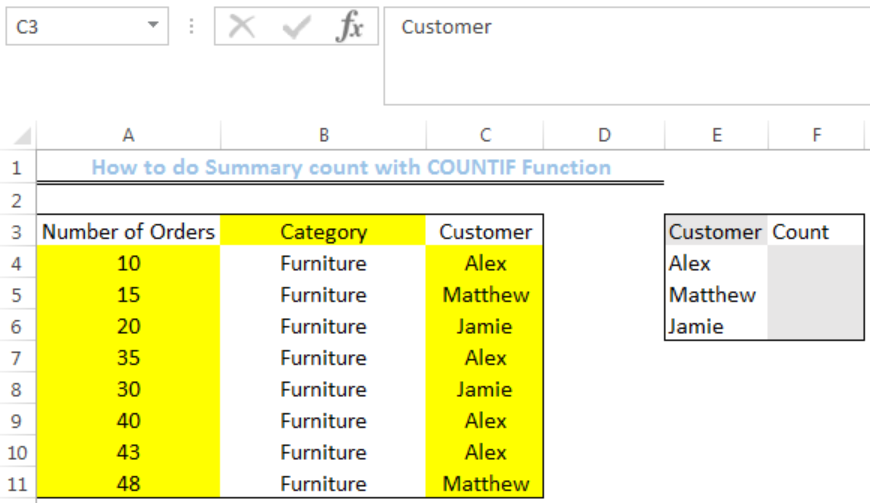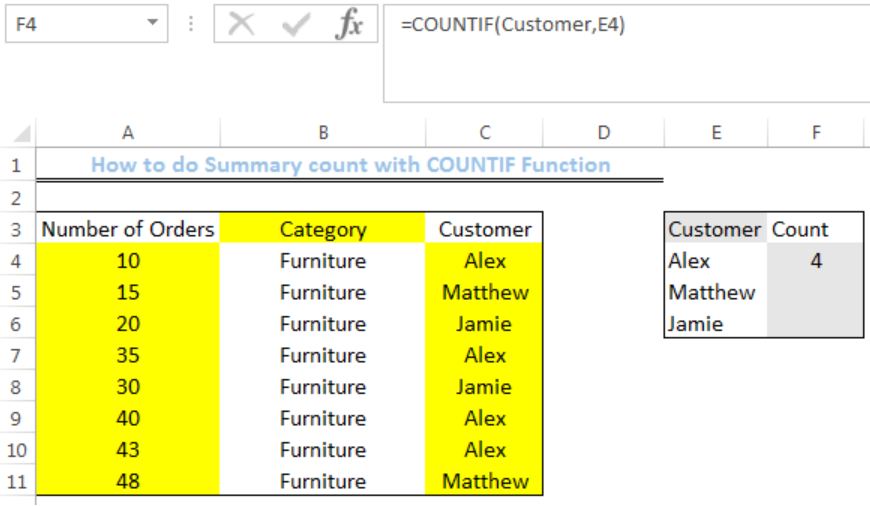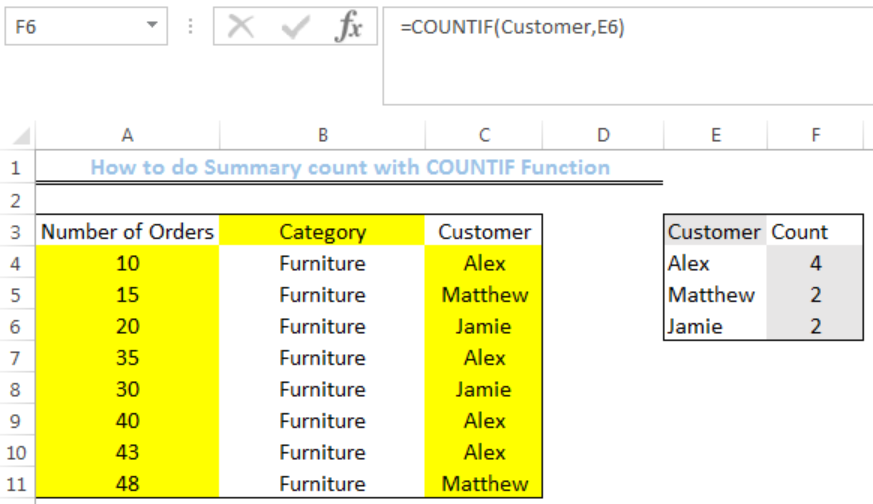Get instant live expert help with Excel or Google Sheets“My Excelchat expert helped me in less than 20 minutes, saving me what would have been 5 hours of work!”

#### Post your problem and you’ll get expert help in seconds.

Your message must be at least 40 characters
Our professional experts are available now. Your privacy is guaranteed.

# How to do Summary Count with COUNTIF Function

We can use the COUNTIF function to count cells within a range that meet a criteria. The steps below will walk through the process.Figure 1: How to do Summary Count with COUNTIF Function

## Syntax

`=COUNTIF(RANGE,CRITERIA)`

• RANGE is the “named range” of items we want a summary count for
• CRITERIA is the cell reference to the specified item

## Formula

`=COUNTIF(Customer,E4)`

## Setting up the Data

• We will set up the data by inputting the Number of orders into Column A
• We will input the category into Column B
• Column C contains the customer name
• Column F is where we want the formula to return the COUNT for each customer
• Note- We will Highlight Cell C4 to Cell C10. We will click on C3 where there is an arrow in figure 2. We will name the range as “CustomerFigure 2: Setting up the Data

## Summary Count with COUNTIF Function

• We will click on Cell F4
• We will insert the formula below into the cell
`=COUNTIF(Customer,E4)`
• We will press the enter keyFigure 3: Summary Count for Alex

• We will click on Cell F4 again
• We will double-click on the fill handle (the small plus sign at the bottom right of Cell F4) and drag down to copy the formula into the other cellsFigure 4: Summary Count for the three Customers

## Explanation

`=COUNTIF(Customer,E4)`

In this formula, the value in Cell E4 is searched for in the named range. COUNTIF returns the sum of the number of times the value appears in the named range.

## Instant Connection to an Expert through our Excelchat Service

Most of the time, the problem you will need to solve will be more complex than a simple application of a formula or function. If you want to save hours of research and frustration, try our live Excelchat service! Our Excel Experts are available 24/7 to answer any Excel question you may have. We guarantee a connection within 30 seconds and a customized solution within 20 minutes.

### Did this post not answer your question? Get a solution from connecting with the expert.Another blog reader asked this question today on Excelchat:
Solution examplesI have a column of percentages (11 in a column). I want to have a percentage at the bottom of the column that tells me how many of the entries are = to 100%.
Solved by X. E. in 20 minsI need a formula to count the number of rows that contain at least one of two possible phrases, but not give me the total number of instances that both phrases occur overall.
Solved by E. B. in 33 minsI want to create a double bar graph from an existing pivot table, but excel won't let me simply add a new field for the column labels and manually put in numbers. What can I do?
Solved by C. F. in 18 minsI have many cells where value is seperated by commas e.g- 1,5,7,8,9 3,0,4,7,8,9,52, Just want to count total value, in the above case it should be 12
Solved by M. J. in 20 minsI have three spreadsheets with names of registered people for an event. I need to combine them and then filter so that I know if any of the people in sheet 1 are also listed on sheet 2 and sheet 3
Solved by C. A. in 40 mins## Subscribe to Excelchat.coAnother blog reader asked this question today on Excelchat: